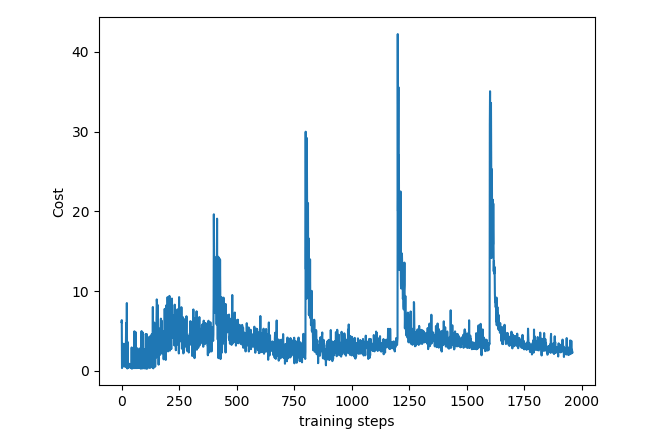## Reinforcement Learning Python DQN Application for Resource Allocation

In the previous post Reinforcement Learning Example for Planning Tasks Using Q Learning and Dyna-Q we applied Dyna-Q algorithm for planning of actions to complete tasks. This problem can be viewed as resource allocation task. In this post we will use reinforcement learning python DQN (Deep Q-network) for the same problem. In case you did not read previous post the problem is described below.

## The Problem

Given some goals (projects to complete) and set of actions (number of hours to put for each project per day) we are interesting to know what action we need to take (how many hours to put per project on each day) in order to get the best result in the end (we have reward for completion project in time).

So we are trying to allocate resource (time) for each project for each day in such way that it produces maximum reward in the end of given period. We have reward data and time needed to complete for each project.

The diagram of one of possible path would look like this:Planning Diagram

On this diagram the green indicates path that produces the max reward 13 as the agent was able to complete both goals.

## Deep Q-Network

Deep Q-Networks, abbreviated DQN, use deep neural networks as function approximation of the
action-value function q(s, a). The input of the artificial neural network used is the state and the output is the estimated q-values of the state-action pairs.

In DQN the replay memory simply stores the transitions such that they can be used at later times. By sampling transitions from the replay memory the network increases its ability to generalize. This also allows the network to predict the correct values in states which might be visited less frequently when the agent’s strategy gets better.

Also we add a second network, a target network, which is a copy of the first network, which we call the training network. The target network is only used to predict the value of taking the optimal action from s0 when updating the training network. The target network is updated with a certain frequency by copying the weights from the training network. This prevents instability when s and s0 are equal or even similar which is often the case. 

## Solution

The code here is based on DQN with Tensorflow for maze problem and previous code for Dyna-Q mentioned in the beginning of the post. It has 2 modules for programming environment and Reinforcement Learning Tensorflow DQN algorithm. Additionally it has main module which run the loop with episodes.

To run this reinforcement learning example you can use reinforcement learning python source code from the links below:

## Results

Below are charts obtained from running program. Performance (achieving max possible reward) with DQN is a little higher (but not significantly) than with Dyna-Q example on the same problem.References

1. Reinforcement learning for planning of a simulated production line Gustaf Ehn, Hugo Werner, February 27, 2018